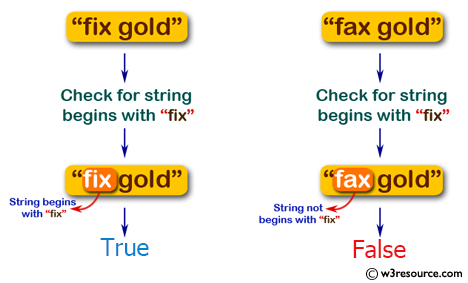﻿ Swift Basic Programming Exercise: Check if a given string begins with "fix", except the 'f' can be any character or number - w3resource# Swift Basic Programming Exercise: Check if a given string begins with "fix", except the 'f' can be any character or number

## Swift Basic Programming: Exercise-13 with Solution

Write a Swift program to check if a given string begins with "fix", except the 'f' can be any character or number.

Pictorial Presentation:Sample Solution:

Swift Code:

``````import Foundation

func fix_Start(_ input: String) -> Bool {
var newInput = input
let startIndex = newInput.startIndex
let first_char = newInput.remove(at: startIndex)

if newInput.hasPrefix("ix") == true
{
newInput.characters.removeFirst(3)
newInput.insert(first_char, at: startIndex)
return true
}
else
{
return false
}
}

print(fix_Start("fix gold"))
print(fix_Start("six gold"))
print(fix_Start("1ix gold"))
print(fix_Start("gold"))
print(fix_Start("fax gold"))
```
```

Sample Output:

```true
true
true
false
false
```

Swift Programming Code Editor:

Improve this sample solution and post your code through Disqus

What is the difficulty level of this exercise?

﻿